Anzeige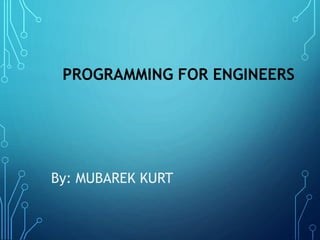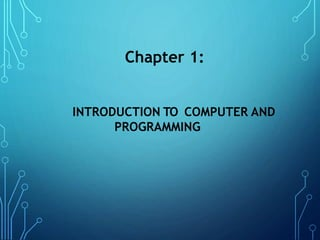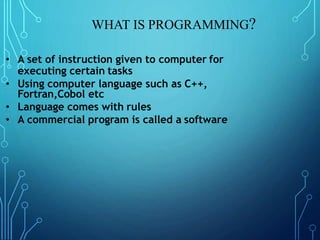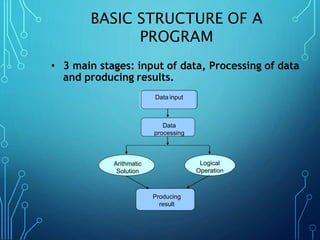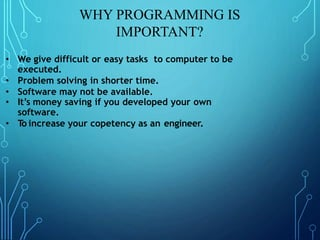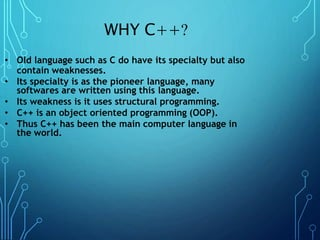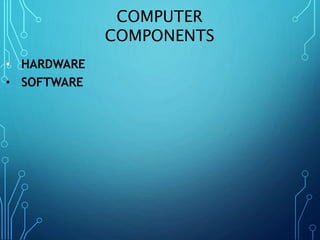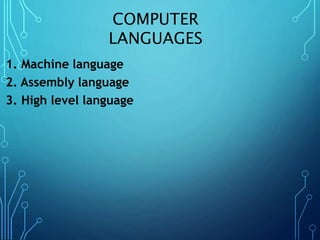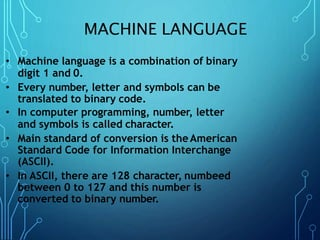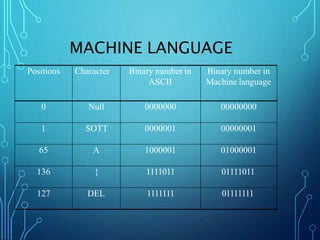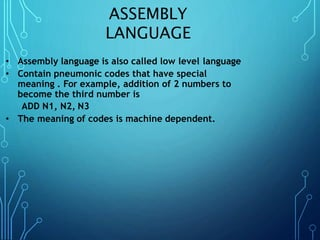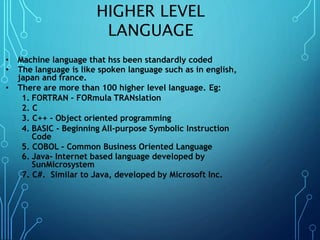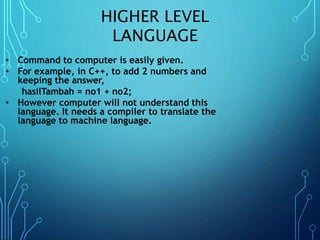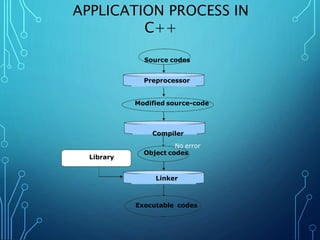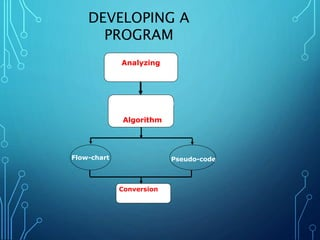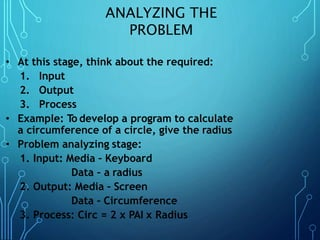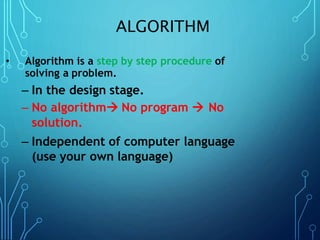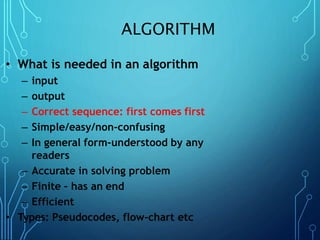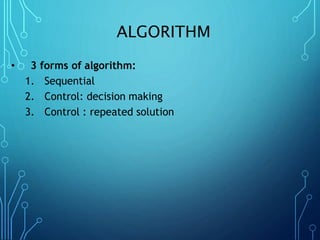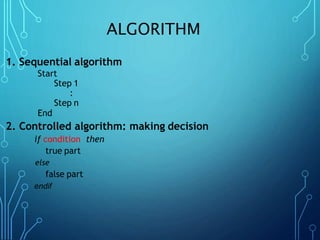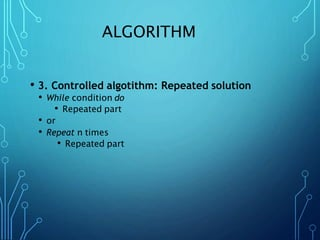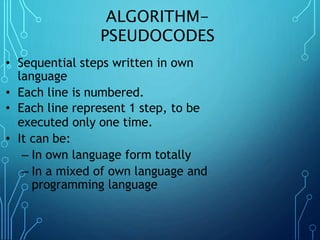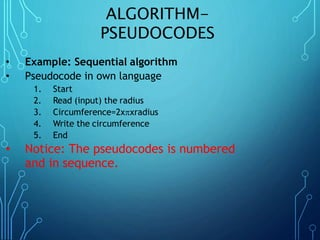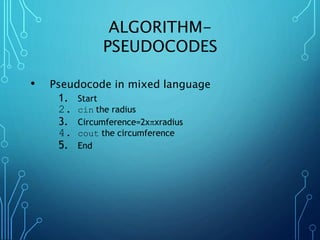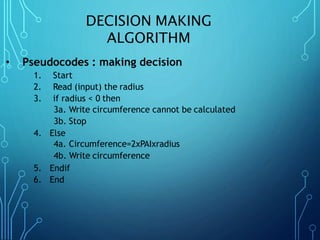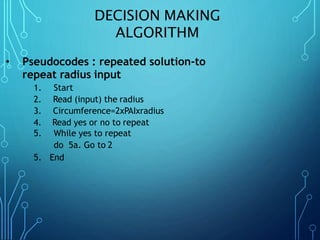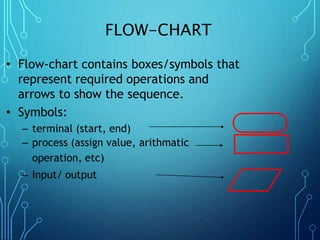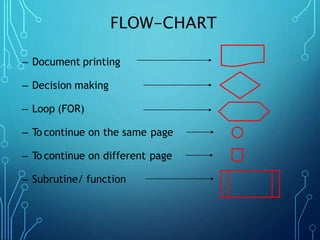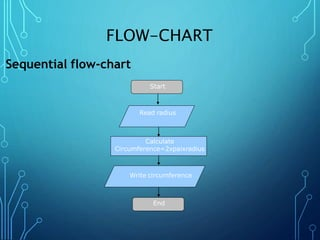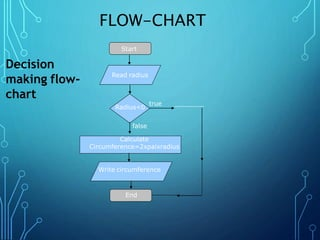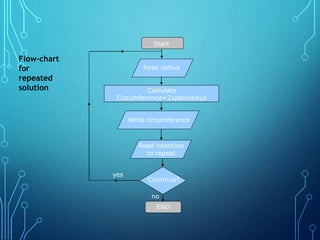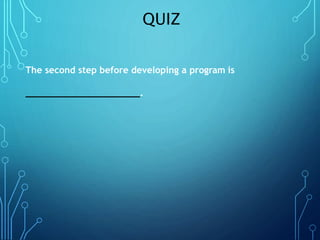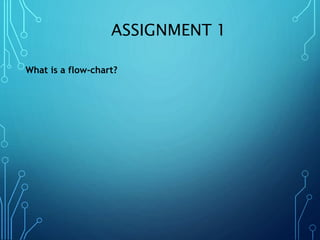1 von 33
Anzeige

### INTRODUCTION TO C++, Chapter 1

1. PROGRAMMING FOR ENGINEERS By: MUBAREK KURT
2. INTRODUCTION TO COMPUTER AND PROGRAMMING Chapter 1:
3. WHAT IS PROGRAMMING? • A set of instruction given to computer for executing certain tasks • Using computer language such as C++, Fortran,Cobol etc • Language comes with rules • A commercial program is called a software
4. BASIC STRUCTURE OF A PROGRAM • 3 main stages: input of data, Processing of data and producing results. Data input Data processing Producing result Arithmatic Solution Logical Operation
5. WHY PROGRAMMING IS IMPORTANT? • We give difficult or easy tasks to computer to be executed. • Problem solving in shorter time. • Software may not be available. • It’s money saving if you developed your own software. • To increase your copetency as an engineer.
6. WHY C++? • Old language such as C do have its specialty but also contain weaknesses. • Its specialty is as the pioneer language, many softwares are written using this language. • Its weakness is it uses structural programming. • C++ is an object oriented programming (OOP). • Thus C++ has been the main computer language in the world.
7. COMPUTER COMPONENTS • HARDWARE • SOFTWARE
8. COMPUTER LANGUAGES 1. Machine language 2. Assembly language 3. High level language
9. MACHINE LANGUAGE • Machine language is a combination of binary digit 1 and 0. • Every number, letter and symbols can be translated to binary code. • In computer programming, number, letter and symbols is called character. • Main standard of conversion is theAmerican Standard Code for Information Interchange (ASCII). • In ASCII, there are 128 character, numbeed between 0 to 127 and this number is converted to binary number.
10. MACHINE LANGUAGE Positions Character Binary number in ASCII Binary number in Machine language 0 Null 0000000 00000000 1 SOTT 0000001 00000001 65 A 1000001 01000001 136 { 1111011 01111011 127 DEL 1111111 01111111
11. ASSEMBLY LANGUAGE • Assembly language is also called low level language • Contain pneumonic codes that have special meaning . For example, addition of 2 numbers to become the third number is ADD N1, N2, N3 • The meaning of codes is machine dependent.
12. HIGHER LEVEL LANGUAGE • Machine language that hss been standardly coded • The language is like spoken language such as in english, japan and france. • There are more than 100 higher level language. Eg: 1. FORTRAN - FORmula TRANslation 2. C 3. C++ - Object oriented programming 4. BASIC - Beginning All-purpose Symbolic Instruction Code 5. COBOL - Common Business Oriented Language 6. Java- Internet based language developed by SunMicrosystem 7. C#. Similar to Java, developed by Microsoft Inc.
13. HIGHER LEVEL LANGUAGE • Command to computer is easily given. • For example, in C++, to add 2 numbers and keeping the answer, hasilTambah = no1 + no2; • However computer will not understand this language. It needs a compiler to translate the language to machine language.
14. APPLICATION PROCESS IN C++ Source codes Preprocessor Modified source-code Compiler No error Object codes Linker Executable codes Library
15. DEVELOPING A PROGRAM Analyzing the problem Program Design using Algorithm Conversion to Program Flow-chart Pseudo-code
16. ANALYZING THE PROBLEM • At this stage, think about the required: 1. Input 2. Output 3. Process • Example: To develop a program to calculate a circumference of a circle, give the radius • Problem analyzing stage: 1. Input: Media – Keyboard Data – a radius 2. Output: Media – Screen Data – Circumference 3. Process: Circ = 2 x PAI x Radius
17. ALGORITHM • Algorithm is a step by step procedure of solving a problem. – In the design stage. – No algorithm No program  No solution. – Independent of computer language (use your own language)
18. ALGORITHM • What is needed in an algorithm – input – output – Correct sequence: first comes first – Simple/easy/non-confusing – In general form-understood by any readers – Accurate in solving problem – Finite – has an end – Efficient • Types: Pseudocodes, flow-chart etc
19. ALGORITHM • 3 forms of algorithm: 1. Sequential 2. Control: decision making 3. Control : repeated solution
20. ALGORITHM 1. Sequential algorithm Start Step 1 : Step n End 2. Controlled algorithm: making decision if condition then true part else false part endif
21. ALGORITHM • 3. Controlled algotithm: Repeated solution • While condition do • Repeated part • or • Repeat n times • Repeated part
22. ALGORITHM- PSEUDOCODES • Sequential steps written in own language • Each line is numbered. • Each line represent 1 step, to be executed only one time. • It can be: – In own language form totally – In a mixed of own language and programming language
23. ALGORITHM- PSEUDOCODES • Example: Sequential algorithm • Pseudocode in own language 1. Start 2. Read (input) the radius 3. Circumference=2xxradius 4. Write the circumference 5. End • Notice: The pseudocodes is numbered and in sequence.
24. ALGORITHM- PSEUDOCODES • Pseudocode in mixed language 1. Start 2. cin the radius 3. Circumference=2xxradius 4. cout the circumference 5. End
25. DECISION MAKING ALGORITHM • Pseudocodes : making decision 1. Start 2. Read (input) the radius 3. if radius < 0 then 3a. Write circumference cannot be calculated 3b. Stop 4. Else 4a. Circumference=2xPAIxradius 4b. Write circumference 5. Endif 6. End
26. DECISION MAKING ALGORITHM • Pseudocodes : repeated solution-to repeat radius input 1. Start 2. Read (input) the radius 3. Circumference=2xPAIxradius 4. Read yes or no to repeat 5. While yes to repeat do 5a. Go to 2 5. End
27. FLOW-CHART • Flow-chart contains boxes/symbols that represent required operations and arrows to show the sequence. • Symbols: – terminal (start, end) – process (assign value, arithmatic operation, etc) – Input/ output
28. FLOW-CHART – Document printing – Decision making – Loop (FOR) – To continue on the same page – To continue on different page – Subrutine/ function